# Weld

The Weld command removes creases from a mesh by merging coincident mesh vertices.

Individual texture mapping, color, and normal vector data is averaged/created/destroyed for the affected vertices.

Weld will create new vertex normals at each welded vertex that is the average of the adjacent face normals. One vertex is shared by more than one face after welding.

Steps

 1 Select a mesh.
 2 Specify the angle tolerance value.

Command-line options

Angle tolerance

If two naked mesh edge points of a selected mesh are coincident, and their neighboring faces are within the angle tolerance of each other, they will be replaced by a single mesh point. If the two points are from different "sub-meshes" of a joined mesh, the meshes become one mesh that cannot explode.

As you change the tolerance, the mesh edges that are within the tolerance, and will therefore be welded, are highlighted.

# WeldEdge

The WeldEdge command removes creases from a mesh by merging coincident mesh vertices along selected edges.

Steps

 1 Select a mesh.
 2 Select edges in the selected mesh.

Command-line options

Undo

Removes the last selected edge.

# WeldVertices

The WeldVertices command removes creases from a mesh by merging all selected mesh vertices.

The WeldVertices command lets you select mesh vertices to weld, rather than the entire mesh. It does not, however, give you a tolerance angle between faces to weld, like the Weld command does. You can window select by turning mesh points on and selecting the points prior to the command or you can run the command and then select multiple individual points.Mesh vertex

The location where the edges of the mesh faces meet. The mesh vertex (plural vertices) contains x-, y-, and z-coordinates and may contain a vector normal, a color value, and texture coordinates.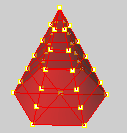Steps

Select mesh vertices, and press Enter.

## Why Weld?

Welding polygon meshes affect rendering, texture mapping on mesh objects, and file export for stereolithography.

### Smoother Rendering

In wireframe these two meshes look the same.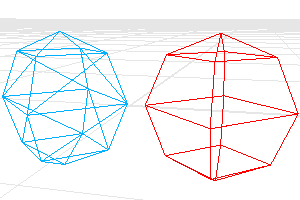When shaded, the red mesh looks smooth and the blue mesh looks chunky.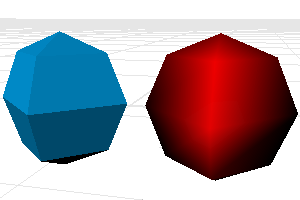Each triangle vertex in the red mesh is welded to its neighbors. This causes the vertices in the red mesh to look smooth. No points in the blue mesh are welded. This causes the vertices in the blue mesh to look sharp.

Suppose you want the blue mesh to look smoother.

First you have to determine the angle tolerance. The shaded creases of this angle should look sharp.

### Texture Mapping

Texture mapping coordinates control how a texture wraps around the object. These are two-dimensional coordinates that are attached to a polygon vertex, and they determine which point on the texture bitmap maps to this vertex. The points between the vertices interpolate from the vertex values.

The bottom left corner of the bitmap is the origin, the bottom right is (1,0), top left is (0,1) and top right is (1,1). The texture mapping coordinates are always between these values.

Only one pair of texture mapping coordinates can attach to a single vertex. That is why if you weld vertices, thus removing the overlapping duplicates, you throw away all but one pair of texture mapping coordinates.

The loss of texture mapping coordinates is irreversible. You cannot recover the mapping coordinates from the remaining mesh information.

To remove welding (and to also lose the texture mapping coordinates), use the UnWeld or the UnWeldEdge command.

### STL Mesh Export Diagnostics

In some rapid prototyping machines, STL files must contain completely closed (watertight) polygon mesh objects.

You might want to use the Join command, then Weld (angle=180), then UnifyMeshNormals to turn a group of meshes into a single watertight mesh object, and use SelNakedMeshEdgePt to find the open (naked) edges. Following this procedure will ensure that the meshes really do fit together before exporting them for use in an expensive STL job.

# Unweld

The Unweld command adds creases to a smooth mesh by creating coincident vertices.

Steps

 1 Select a mesh.
 2 Specify the angle tolerance value.

Command-line options

ModifyNormals=Yes/No

If Yes, the vertex normals on each side of the edge take the same value as the face to which they belong, giving the mesh a hard edge look.

If No, each of the vertex normals on either side of the edge is assigned the same value as the original normal that the pair is replacing, keeping a smooth look.

# UnweldEdge

The UnweldEdge command adds creases to a smooth mesh by creating coincident vertices along selected edges.Steps

 1 Select a mesh.
 2 Select edges in the selected mesh.

Command-line option

ModifyNormals=Yes/No

If Yes, the vertex normals on each side of the edge take the same value as the face to which they belong, giving the mesh a hard edge look.

If No, each of the vertex normals on either side of the edge is assigned the same value as the original normal that the pair is replacing, keeping a smooth look.

## What welding means

Internally, a mesh is defined by two lists:

 ● A list of vertices and their attributes (position, normal, texture-coordinates, vertex-color).
 ● A list of face definitions describing how these vertices are connected to form faces.

## Examples

This two-part definition describes two quadrangular faces that do not share vertices as shown in the illustration.

Vertex list

The vertex list is half of the mesh definition, describing the vertices.Note: In this example texture coordinates and vertex colors are left out, but those are per-vertex attributes as well.

vertex 1 : location, normal

vertex 2 : location, normal

vertex 3 : location, normal

vertex 4 : location, normal

vertex 5 : location, normal

vertex 6 : location, normal

vertex 7 : location, normal

vertex 8 : location, normal

Face definitions

This list describes how these vertices are connected to form faces.

face 1 : vertex 1, vertex 2, vertex 3, vertex 4

face 2 : vertex 5, vertex 6, vertex 7, vertex 8

Triangular mesh example 1

A mesh with a list like the following example describes two separate triangular faces, not touching each other, as shown in the illustration.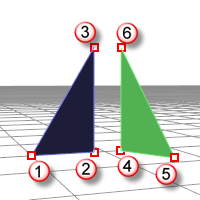vertex 1 : location, normal

vertex 2 : location, normal

vertex 3 : location, normal

vertex 4 : location, normal

vertex 5 : location, normal

vertex 6 : location, normal

face 1 : vertex 1, vertex 2, vertex 3

face 2 : vertex 4, vertex 5, vertex 6

Triangular mesh example 2

In the next example, imagine two of those six vertices share the same position: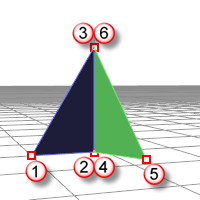vertex 1 : location, normal

vertex 2 : location, normal

vertex 3 : location, normal

vertex 4 : location, normal

vertex 5 : location, normal

vertex 6 : location, normal

face 1 : vertex 1, vertex 2, vertex 3

face 2 : vertex 4, vertex 5, vertex 6

Here vertices 2 and 4 are at the same position and 3 and 6 are at the same position.

So we have a mesh consisting of two faces that share one edge, but vertices 2, 3, 4 and 6 all have their own individual attributes. So their normals can be different.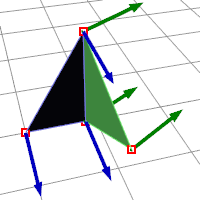Joined mesh

When you Join a mesh, the vertices and faces are in no way merged; the lists are simply appended. The face normals retain their direction.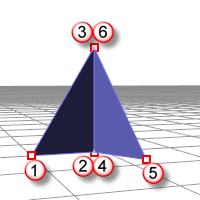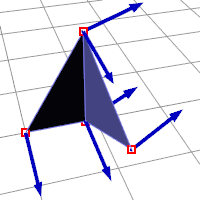Welded mesh

When you Weld a mesh and the angle between the faces is below the threshold the common edge will be welded.

The two common vertices are replaced by a new vertex with new attributes:vertex 1 : location, normal

vertex 5 : location, normal

vertex 7 : location, normal

vertex 8 : location, normal

face 1 : vertex 1, vertex 7, vertex 8

face 2 : vertex 7, vertex 5, vertex 8

New normal attributes for vertices 7 and 8 are averaged based on the normals of the faces they are part of, so there is no way to get the previous (pre-weld) normals back.Summary

 ● Joining does not destroy any vertex attributes.
 ● Welding "merges" vertices on common edges below the angle threshold.
 ● The resulting new vertex has it's normal calculated based on the average of the faces it belongs to.